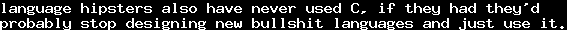# InductionSun, 28 Jun 2015 15:32:53 GMT

Induction is one of the nightmares of many computer science students, it seems. For mathematicians, it is a very natural concept, and often the first higher proof strategy. Induction itself is not hard, the problem is that most proofs involve a lot more work than just induction, and it can be hard to even find the right parameters for induction.

When doing type theory, one quickly notices that induction is somehow equivalent to recursion: Recursion operators have a type signature similar to the formulas which encode induction principles. This is not a coincidence - but recursion operators are not really intuitive either, for a beginner. However, I think that for many beginners who have at least a little knowledge about programming, the key for understanding induction could be recursion. Even though from a purely "practical" viewpoint, recursion might not be good programming practice (especially if you ask older programmers), it is a well-understood concept.

I assume that the reader knows what a function - in the sense of programming - is. I will use C notation here, but without pointer magic, to make it easily understandable.

A well known function is the factorial of a number. Mathematical notation for the factorial ofis usually. For, we can "define" the factorial as. Furthermore, it is defined that. A simple implementation of this function in C would look like

int fact(int n) {
int ret = 1;
for (int i = 1; i <= n; i++) {
ret = ret * i;
}
return ret;
}


And in fact, this would calculate the factorial (and is probably the way most software engineers would recommend). On the other hand, notice thatfor. This equation andfully determine the values of the factorial function. So, an alternative way to write a factorial function in C is

int fact (int n) {
if (n == 0) return 1;
else return n * fact(n - 1);
}


This function calls itself. This is called recursion, the function is called recursive. Notice that the function argument decreases. Behind this lies a simple principle: We can already calculate the function for a smaller argument, and we can use this value to calculate the function's value for the larger argument. We can reduce the problem to a simpler problem.

Another classical example for recursion (and especially for the problems with recursion) is the Fibonacci sequence. We defineandfor. This is a recursive definition. In C, we can write

int fib (int n) {
if (n < 3) {
return 1;
} else {
return fib(n-1) + fib(n-2)
}
}


This definition has a major disadvantage: Its runtime is exponential (if you don't know what that means: really really slow). But it also has a great advantage: It is easy to write and easy to understand. The non-recursive definition looks like

int fib (int n) {
int j = 1, k = 1;
for (int i = 3; i <= n; i++) {
int l = j + k;
j = k;
k = l;
}
return k;
}


This function is (arguably) harder to understand. This is often the case, and this is a common problem that computer science tries to solve: Things which are easy for humans are hard for computers and vice versa. That is why modern programming languages have abstractions like functions, classes, modules, etc. The art of computer science is to find a common language that both computers and humans understand well. For the hardest parts, people rely on mathematical proofs.

And one principle that makes it easier for humans to reason is to reduce a problem on simpler problems. And this is what recursion can do.

Now back to induction. The classical introduction to induction usually contains Gaussian sums: Assume we want to add the numbers fromto, that is, we want to calculate. This is similar to the factorial, except that we use addition instead of multiplication. And of course, we can use the same function as above, and just replace multiplications with additions (and 1 with 0):

int gauss (int n) {
if (n == 0) return 0;
else return n + gauss(n - 1);
}


This function loops through all numbers below n, it takes linear time. This is not bad, but there is an easier way:

int gauss (int n) {
return (n * (n + 1))/2;
}


In fact,for all. How do we know that this holds for every natural number? We can calculate this for every single number, but not for all numbers, as there are infinitely many natural numbers: We need a mathematical proof.

Before we go into induction, let us define a function from natural numbers to proofs. It is possible to do this formally, but not without a deeper understanding of mathematical logic - so we just do this informally here. Let us call this function. That is,is a proof for,is a proof for, and in general,is a proof for. We will define this function recursively.

For, we can just calculate this. So, we can say.

Forwith, we can do recursion.proves that. Using this proof, we can setWe recursively defined a function that generates proofs. We did this in a very informal way, but with just a little more theory, we could do this in a fully formal way. (If you're interested, look at proof assistants like Coq or Agda.)

Denote byour proposition. Notice thatis a proof of.

Notice that our definition ofproves. Our definition offor the other cases uses- a proof of- to prove. So essentially, one could say that the recursive definition is itself a proof of. And notice that these are the premises of induction: Ifand for allwe have, then we havefor all.

There are more general induction schemes. For example, on binary trees, you can do induction on every branch - but the same way you can do recursion. The important part about induction (and recursion) is that something decreases, which cannot decrease infinitely often.